## Angles and Angle Pairs

Easily as significant as rays and line segments are the angles they form. Without them, there would be none of the geometric figures that you know (with the possible exception of the circle).

## Angles

Two rays that have the same endpoint form an angle. That endpoint is called the vertex, and the rays are called the sides of the angle. In geometry, an angle is measured in degrees from 0° to 180°. The number of degrees indicates the size of the angle. In Figure 1, rays AB and AC form the angle. A is the vertex.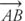and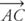are the sides of the angle.Figure 1 ∠BAC.

The symbol ∠ is used to denote an angle. The symbol m ∠ is sometimes used to denote the measure of an angle.

An angle can be named in various ways (Figure 2).Figure 2 Different names for the same angle.

• By the letter of the vertex—therefore, the angle in Figure could be named ∠ A.
• By the number (or small letter) in its interior—therefore, the angle in Figure could be named ∠1 or ∠ x.
• By the letters of three points that form it—therefore, the angle in Figure could be named ∠ BAC or ∠ CAB. The center letter is always the letter of the vertex.

Example 1: In Figure 3(a) use three letters to rename ∠3; (b) use one number to rename ∠ KMJ.Figure 3 Different names for the same angle

(a) ∠3 is the same as ∠ IMJ or ∠ JMI;

(b) ∠ KMJ is the same as ∠ 4.

Postulate 9 (Protractor Postulate): Suppose O is a point on. Consider all rays with endpoint O that lie on one side of. Each ray can be paired with exactly one real number between 0° and 180°, as shown in Figure 4. The positive difference between two numbers representing two different rays is the measure of the angle whose sides are the two rays.Figure 4 Using the Protractor PostulateExample 2: Use Figure 5 to find the following: (a) mSON, (b) mROT, and (c) mMOE.Figure 5 Using the Protractor Postulate.

• (a)

mSON = 40° −0°

mSON = 40°

• (b)

mROT = 160° −70°

mROT = 90°

• (c)

mMOE = 180° −105°

mMOE = 75°

Postulate 10 (Angle Addition Postulate): Iflies between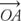and, then mAOB + mBOC = mAOC (Figure 6).Example 3: In Figure 7, if m ∠1 = 32° and m ∠2 = 45°, find mNEC.Because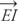is between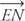and, by Postulate 10,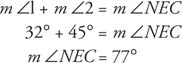## Angle bisector

An angle bisector is a ray that divides an angle into two equal angles. In Figure 8,is a bisector of ∠ XOZ because = mXOY = mYOZ.Figure 8 Bisector of an angle

Theorem 5: An angle that is not a straight angle has exactly one bisector.

Certain angles are given special names based on their measures.

## Right angle

A right angle has a measure of 90°. The symbolin the interior of an angle designates the fact that a right angle is formed. In Figure 9, ∠ ABC is a right angle.Figure 9 A right angle.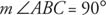Theorem 6: All right angles are equal.

## Acute angle

An acute angle is any angle whose measure is less than 90°. In Figure 10, ∠ b is acute.Figure 10 An acute angle.## Obtuse angle

An obtuse angle is an angle whose measure is more than 90° but less than 180°. In Figure 11 , ∠4 is obtuse.Figure 11 An obtuse angle.## Straight angle

Some geometry texts refer to an angle with a measure of 180° as a straight angle. In Figure 12, ∠ BAC is a straight angle.Figure 12 A straight angleExample 4: Use Figure 13 to identify each named angle as acute, right, obtuse, or straight: (a) ∠ BFD, (b) ∠ AFE, (c) ∠ BFC, (d) ∠ DFA.Figure 13 Classification of angles

• (a)

mBFD = 90° (130° − 40° = 90°), so ∠ BFD is a right angle.

• (b)

mAFE = 180°, so ∠ AFE is a straight angle.

• (c)

mBFC = 40° (130° − 90° = 40°), so ∠ BFC is an acute angle.

• (d)

mDFA = 140° ( 180° − 40° = 140°), so ∠ DFA is an obtuse angle.